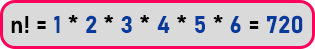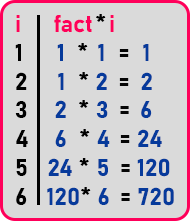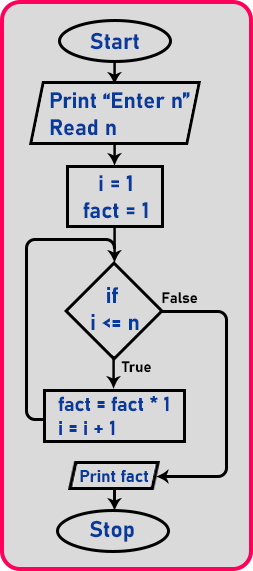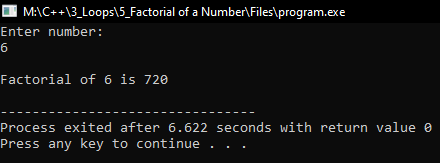# Factorial of a Number using Loop in C++

## Factorial of a Number using Loop in C++

In this article, I am going to discuss the Program to Print Factorial of a Number using Loop in C++ with Examples. Please read our previous articles, where we discussed the Sum of N natural numbers using loop in C++ with Examples.

##### Factorial of a Number:

Let us understand the procedure then the flowchart and then the program. If we have a number ‘n = 6’, integer number, it should not be a decimal number. Factorial of that number means the product of first ‘n’ natural numbers that areThat is factorial. So, 6! = 720. Here, we are multiplying numbers i.e. 1 * 2 * … up to that number which we want factorial. Multiplication is a repeating step, when it is repeating then we have to do it by using a loop. So how it is repeated? To understand that we have data below in the form of a table. Let us explain these step by step.1. First, we multiply ‘1’ with ‘1’, because there is no other number, so the result is ‘1’. If we multiply with ‘0’ then the result of the factorial will be zero.
2. Now, multiply ‘2’ with the previous result that is ‘1 * 2 = 2’.
3. Now, again multiply ‘3’ to the result of previous multiplication ‘2 * 3 = 6’.
4. Multiply ‘4’ with the previous result that is ‘6 * 4 = 24’.
5. Multiply ‘5’ with the result of the previous addition that is ’24 * 5 = 120’.
6. Multiply ‘6’ to the previous result that is ’120 * 6 = 720’.

The result of ‘6!’ is 720. In this way, we can calculate the factorial of any number. So ‘i’ is multiplied with the value in every step. And the result is stored in that value. So let us call this value ‘fact’. And in each step, we are multiplying this ‘fact’ value with ‘i’ as ‘fact = fact * i’. So, we are modifying ‘fact’ in each and every step. So initially the value of ‘fact’ is ‘1’. Now let us look at the flowchart:

##### Factorial of a Number Flowchart:Let us study that flow chart. First, we take a number from the user. Then we should initialize the counter to ‘1’ as well as our ‘fact’ variable to ‘1’, we will not initialize ‘fact’ to ‘0’ as the result will be 0. Both ‘i’ and ‘fact’ will be modified in every iteration of the loop.

Then up to where we want to find the factorial, let’s take that number be ‘n’. So, we will check the condition as ‘if (i <= n)’. If our counter is equal to the given number then the loop will be exit.

If the condition will true then modify the ‘fact’ variable as ‘fact = fact * i’ and increment the ‘i’ variable by 1. After this step, control goes back to the condition and check if the condition is true then again modify the ‘sum’ and ‘i’ variables and it will continue until the condition become false. If the condition will false, then simply stop the loop. Now let us look at the program.

##### Program to find Factorial of a number using loop in C++:
```#include <iostream>
using namespace std;
int main()
{
int n, fact = 1;
cout << "Enter number:" << endl;
cin >> n;
cout << endl;
for (int i = 1; i <= n; i++)
{
fact *= i;
}
cout << "Factorial of " << n << " is " << fact << endl;
return 0;
}
```
###### Output: